Courses

# Signals And Systems 2 - MCQ Test 2

## 20 Questions MCQ Test Mock Test Series for Electrical Engineering (EE) GATE 2020 | Signals And Systems 2 - MCQ Test 2

Description
This mock test of Signals And Systems 2 - MCQ Test 2 for GATE helps you for every GATE entrance exam. This contains 20 Multiple Choice Questions for GATE Signals And Systems 2 - MCQ Test 2 (mcq) to study with solutions a complete question bank. The solved questions answers in this Signals And Systems 2 - MCQ Test 2 quiz give you a good mix of easy questions and tough questions. GATE students definitely take this Signals And Systems 2 - MCQ Test 2 exercise for a better result in the exam. You can find other Signals And Systems 2 - MCQ Test 2 extra questions, long questions & short questions for GATE on EduRev as well by searching above.
QUESTION: 1

Solution:
QUESTION: 2

Solution:
QUESTION: 3

### The Laplace transform of the signal f(t) = 2e-at sin (bt +θ) is equal to

Solution:
QUESTION: 4

consider a square pulse f(t) as shown in figure below. Let F(s) denote the Laplace transform of f(t)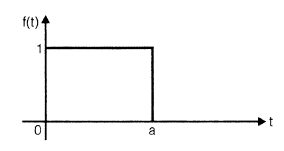then the resulting wave form of the inverse Laplace Transform of F2(s) is

Solution:
QUESTION: 5

The Laplace Transform of the signal x(t) = (t2 - 2t) u(t - 1)

Solution:
QUESTION: 6

Consider a LTI system with system function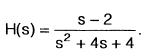The steady-state response of the system is given by (when the excitation is 8 cos2t)

Solution:
QUESTION: 7

The Laplace transform of the signal x(t) whose waveform is shown below is given by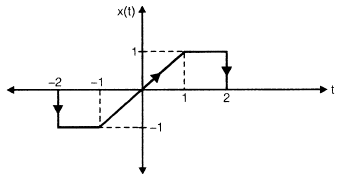Solution:
QUESTION: 8

The inverse z-transform of a z-domain funcion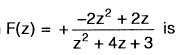Solution:
QUESTION: 9

A discrete-time system is given by the equation

y(k + 3) = -0.3y(k + 2) + y(k + 1) - 0.5y(k) + 2x(k + 3) - 4x(k + 1)

The highest degree of ‘z’ in Numerator and Denominator polynomials of its transfer function H(z) will be, (Assume all initial conditions are zero).

Solution:
QUESTION: 10

Consider the discrete time sequence,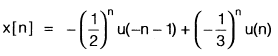and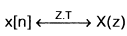have some ROCs.

1. The ROC of X(z) constains the unit circle.
2. The ROC of X(z) does not contains the unit circle.
3. The discrete sequence x[n], does not have a Fourier transform.
4. The discrete sequence x[n] does have a Fourier transform.

Q.

Which is/are correct statement(s)?

Solution:
QUESTION: 11

If the impulse response of discrete-time sequence is h[n] = an • u[-n - 1], then the system function H(z) equals to, (Assume that α = 1.6)

Solution:
QUESTION: 12

The z-transform X(z) of a discrete-time sequence x[n] is equals to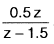It is given that the ROC of X(z) does not includes the unit circle, u[n] = 1 for n ≥ 0. The value of x[n] at n = 1 equals to

Solution:
QUESTION: 13

Consider a general signal x(t) which is sampled at sampling rate 1/T to get the discrete-time sequence x(kT) having z-transform X(z). Assume X(s) is the Laplace transform of x(t). For X(s) = X(z), which is the correct relation between s and z?

Solution:
QUESTION: 14

A system is described by the difference equation,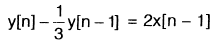The impulse response of the system is

Solution:
QUESTION: 15

Consider a waveform f(t)as shown in figure below: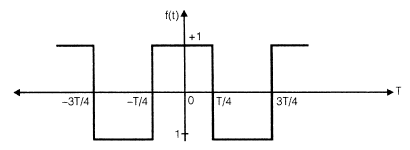The Fourier series of f(t) have

Solution:
QUESTION: 16

The Fourier series expansion of a periodic function f(t) contains only odd harmonics of sine waves. It is clearly say that funciton f(t) is an

Solution:
QUESTION: 17

If X(f) represents the Fourier transform of a signal x(t), which is real and odd symmetric in time, then

Solution:
QUESTION: 18

A signal x(t) has fourier transform X(ω) which is shown in the figure below: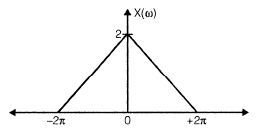The value present in the signal x(t) at t =0 is

Solution:
QUESTION: 19

A source signal s(t) having a Fourier transform as,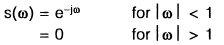The energy and the power that would be observed through a 1 Ω resistor respectively will be

Solution:
QUESTION: 20

Assuming x(t) is an even function. Then

Fourier transform of x(t) will be:

Solution: